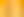The Art of Angles

# Stages1. Playing with Polygons

EXPLORE

Suggested Learning Intentions

• To develop vocabulary about the angle and side properties of triangles and quadrilaterals
• To classify triangles according to their side and angle properties and describe quadrilaterals

Sample Success Criteria

• I can describe a polygon using its properties
• I can use side and angle properties of triangles and quadrilaterals to describe their differences and similarities
• I can identify polygons and non-polygons within a two-dimensional image
• I can explain and justify my thinking using manipulatives
EXPLORE

EXPLORE

Suggested Learning Intentions

• To investigate the way that angles in shapes relate to each other
• To manipulate angles to improve understanding of angle properties in polygons

Sample Success Criteria

• I can estimate the size of an angle when turning an object
• I can identify polygons and angles made when lines cross
• I can prove the sum of the internal angles in a triangle or quadrilateral
• I can use a range of manipulatives to explain and justify my solutions
EXPLORE

3. Dancing Between the Lines

EXPLORE

Suggested Learning Intentions

• To explore the properties of angles where a transversal crosses parallel lines
• To make connections between line and angle forms in artwork and line and angle properties in mathematics
• To use properties of lines and angles to discuss visual elements in artworks

Sample Success Criteria

• I can identify angles using mathematical language used in relation to a transversal crossing parallel lines
• I can use technology to create visual representations of angle problems
• I can identify parallel lines in two-dimensional images
EXPLORE

4. Untangle Polygons and Angles

EXPLORE

Suggested Learning Intentions

• To develop logical reasoning to solve problems involving lines, angles, and polygons
• To articulate mathematical reasoning through providing justifications of steps in problem solving
• To use visual representations to support problem solving

Sample Success Criteria

• I can provide justifications for mathematical reasoning when problem solving
• I can use a range of manipulatives to explain and justify my thinking
• I can match justifications to a visual representation of a problem
• I can reference mathematical facts when proving an answer in problem solving
EXPLORE